# Creating and using structures

### Creating structures

In ColdFusion, you can create structures explicitly by using a function, and then populate the structure using assignment statements or functions, or you can create the structure implicitly by using an assignment statement.

### Creating structures using functions

You can create structures by assigning a variable name to the structure with the StructNew function as follows:

```<cfscript>
structName=StructNew();
</cfscript>```

For example, to create a structure named departments, use the following syntax:

```<cfscript>
departments=StructNew();
</cfscript>```

This statement creates an empty structure to which you can add data.

### Support for ordered structs

In the 2016 release of ColdFusion, you can create a struct that maintains insertion order.

When you loop over the struct using StructNew(“Ordered”), keys are fetched according to the insertion order.

For example,

```<cfscript>
departments = structNew("Ordered");
/** On iterating this struct, you get the values in insertion order, which is the way you inserted the values. **/
/** Create a structure and set its contents. **/

departments.John = "Sales";
departments.Tom = "Finance";
departments.Mike = "Education";
departments.Andrew = "Marketing";

/** Build a table to display the contents **/

<tr>
<td><b>Employee</b></td>
<td><b>Department</b></td>
</tr>");
//  Use cfloop to loop through the departments structure.The item attribute specifies a name for the structure key.
for ( person in departments ) {

writeOutput("<tr>
<td>#person#</td>
<td>#Departments[person]#</td>
</tr>");
}

writeOutput("</table>");
</cfscript>```

The code produces the following output:

Employee

Department

John

Sales

Tom

Finance

Mike

Education

Andrew

Marketing

### Creating structures implicitly

In the 2016 release of ColdFusion, you can create an empty ordered structure implicitly, as in the following example:

<cfset myStruct = [:]> OR <cfset myStruct = [=]>

The following example shows how to create struct from literal syntax:

departments = [Marketing = "John", Sales : [Executive : "Tom", Assistant = "Mike"]];

You can create an empty structure implicitly, as in the following example:

```<cfscript>
myStruct = {};
</cfscript>```

You can also create a structure by assigning data to a variable. For example, each of the following lines creates a structure named myStruct with one element, name, that has the value Adobe Systems Incorporated.

```<cfscript>
coInfo=StructNew();
</cfscript>```

When you use structure notation to create a structure, as shown in the third example, you can populate multiple structure fields. The following example shows this use:

```<cfscript>
</cfscript>```

ColdFusion allows nested implicit creation of structures, arrays, or structures and arrays. For example,

```<cfscript>
myStruct = {structKey1 = {innerStructKey1 = "innerStructValue1"}};
</cfscript>
```

You can use object .property notation on the left side of assignments inside structure notation. For example,

```<cfscript>
myStruct={structKey1.innerStructKey1 = "innerStructValue1"};
</cfscript>```

You can also use multiple statements, such as the following:

```<cfscript>
innerStruct1 = {innerStructKey1 = "innerStructValue1"};
myStruct1={structKey1 = innerStruct1};
</cfscript>```

You cannot use a dynamic variable when you create a structure implicitly. For example, the following expression generates an error:

```<cfscript>
i="coInfo";
</cfscript>```

### Using implicitly created structures in functions and tags

You can use implicitly created structures directly in functions (including user-defined functions) and tags. For example, the following code dumps an implicitly created structure.

```<cfscript>
writeDump( var={Name ="28 Weeks Later", Time = "7:45 PM"} );
</cfscript>```

You can use array notation inside the structure notation, as shown in the following example:

```<cfscript>
student = {firstName="Jane", lastName="Janes", grades=[91, 78, 87]};
</cfscript>```

### Adding and updating structure elements

You add or update a structure element to a structure by assigning the element a value or by using a ColdFusion function. It is simpler and more efficient to use direct assignment.
You can add structure key-value pairs by defining the value of the structure key, as the following example shows:

```<cfscript>
myStruct=StructNew();
myStruct.key1="A new structure with a new key";
WriteDump(myStruct);
myStruct.key2="Now I've added a second key";
WriteDump(myStruct);
</cfscript>```

The following code uses cfset and object.property notation to create a structure element called departments.John, and changes John's department from Sales to Marketing. It then uses associative array notation to change his department to Facilities. Each time the department changes, it displays the results:

```<cfscript>
departments=structnew();
departments.John = "Sales";
writeOutput("Before the first change, John was in the #departments.John# Department");
Departments.John = "Marketing";
writeOutput("After the first change, John is in the #departments.John# Department");
Departments["John"] = "Facilities";
writeOutput("After the second change, John is in the #departments.John# Department");
</cfscript>```

### Getting information about structures and keys

You use ColdFusion functions to find information about structures and their keys.

To find out if a given value represents a structure, use the IsStruct function, as follows:

`IsStruct(variable)`

This function returns True if variable is a ColdFusion structure. (It also returns True if variable is a Java object that implements the java.util.Map interface.)

Structures are not indexed numerically, so to find out how many name-value pairs exist in a structure, use the StructCount function, as in the following example:

`StructCount(employee)`

To discover whether a specific Structure contains data, use the StructIsEmpty function, as follows:

`StructIsEmpty(structure_name)`

This function returns True if the structure is empty, and False if it contains data.

### Finding a specific key and its value

To determine whether a specific key exists in a structure, use the StructKeyExists function, as follows:

`StructKeyExists(structure_name, "key_name")`

Do not place the name of the structure in quotation marks, but you do place the key name in quotation marks.

```if ( StructKeyExists(myStruct, "myKey") ) {
cfoutput(  ) {

writeOutput("#mystruct.myKey#");
}
}
```

You can use the StructKeyExists function to dynamically test for keys by using a variable to represent the key name. In this case, you do not place the variable in quotation marks. For example, the following code loops through the records of the GetEmployees query and tests the myStruct structure for a key that matches theLastName field of the query. If ColdFusion finds a matching key, it displays the Last Name from the query and the corresponding entry in the structure.

```<cfloop query="GetEmployees">
<cfif StructKeyExists(myStruct, LastName)>
<cfoutput>#LastName#: #mystruct[LastName]#</cfoutput><br>
</cfif>
</cfloop>```

If the name of the key is known in advance, you can also use the ColdFusion IsDefined function, as follows:

`IsDefined("structure_name.key")>`

However, if the key is dynamic, or contains special characters, use the StructKeyExists function.

Hinweis:

Using StructKeyExists to test for the existence of a structure entry is more efficient than using IsDefined. ColdFusion scopes are available as structures and you can improve efficiency by using StructKeyExists to test for the existence of variables.

### Getting a list of keys in a structure

To get a list of the keys in a CFML structure, you use the StructKeyList function, as follows:

```<cfscript>
temp=StructKeyList(structure_name, [delimiter]);
</cfscript>```

### Example of using StructKeyList

```<cfscript>
myStruct=StructNew();
myStruct.key1="Bugatti";
myStruct.key2="Lamborghini";
myStruct.key3="Maserati";
myStruct.key4="Ferrari";
myStruct.key5="Aprilia";
myStruct.key5="Ducati";
WriteOutput("The input struct is:");
WriteDump(myStruct);
WriteOutput("struct has " & StructCount(myStruct) & " keys: " & StructKeyList(myStruct) & "<br/>");
</cfscript>```

You can specify any character as the delimiter; the default is a comma.

Use the StructKeyArray function to returns an array of keys in a structure, as follows:

```<cfscript>
temp=StructKeyArray(structure_name);
</cfscript>```
Hinweis:

The StructKeyList and StructKeyArray functions do not return keys in any particular order. Use the ListSort or ArraySort functions to sort the results.

### Copying structures

ColdFusion provides several ways to copy structures and create structure references. The following table lists these methods and describes their uses:

Technique

Use

Duplicate function

Makes a complete copy of the structure. All data is copied from the original structure to the new structure, including the contents of structures, queries, and other objects. As a result changes to one copy of the structure have no effect on the other structure.This function is useful when you want to move a structure completely into a new scope. In particular, if a structure is created in a scope that requires locking (for example, Application), you can duplicate it into a scope that does not require locking (for example, Request), and then delete it in the scope that requires locking.

StructCopy function

Makes a shallow copy of a structure. It creates a structure and copies all simple variable and array values at the top level of the original structure to the new structure. However, it does not make copies of any structures, queries, or other objects that the original structure contains, or of any data inside these objects. Instead, it creates a reference in the new structure to the objects in the original structure. As a result, any change to these objects in one structure also changes the corresponding objects in the copied structure.The Duplicate function replaces this function for most, if not all, purposes.

Variable assignment

Creates an additional reference, or alias, to the structure. Any change to the data using one variable name changes the structure that you access using the other variable name.This technique is useful when you want to add a local variable to another scope or otherwise change the scope of a variable without deleting the variable from the original scope.

### Copying a structure

```<cfscript>
myStruct = StructNew();
myNewStruct = StructNew();
myStruct.key1 = "The quick brown fox";
myStruct.key2 = "jumped over the";
myStruct.key3 = "lazy dog";
myNewStruct.k1 = "Atlantic";
myNewStruct.k2 = "Pacific";
myNewStruct.k3 = "Indian";
myArray="North Island";
myArray="South Island";
myStruct.key4 = myNewStruct;
// Assign myArray as a key to myNewStruct
myNewStruct.k4=myArray;
// Print myStruct
WriteOutput("The original struct is:");
WriteDump(myStruct);
// Copy the structure myStruct into a structure called copiedStruct
copiedStruct=StructCopy(myStruct);
// Print copiedStruct
WriteOutput("The copied struct is:");
WriteDump(copiedStruct);
</cfscript>```

### Duplicating a structure

```<cfscript>
myStruct = StructNew();
myNewStruct = StructNew();
myStruct.key1 = "The quick brown fox";
myStruct.key2 = "jumped over the";
myStruct.key3 = "lazy dog";
myNewStruct.k1 = "Atlantic";
myNewStruct.k2 = "Pacific";
myNewStruct.k3 = "Indian";
myArray="North Island";
myArray="South Island";
myStruct.key4 = myNewStruct;
// Assign myArray as a key to myNewStruct
myNewStruct.k4=myArray;
// Print myStruct
WriteOutput("The original struct is:");
WriteDump(myStruct);
// copy the structure myStruct into a structure called copiedStruct
copiedStruct=StructCopy(myStruct);
// Change copiedStruct properties
copiedStruct.n1="Alien";
copiedStruct.n2="Predator";
copiedStruct.n3="Terminator";
// Create a new struct anotherStruct
anotherStruct=StructNew();
anotherStruct.t1="Amazon";
anotherStruct.t2="Nile";
anotherStruct.t3="Danube";
// Assign anotherStruct as a new key to copiedStruct
copiedStruct.n4=anotherStruct;
// Print copiedStruct
WriteOutput("The changed struct,copiedStruct, is:");
WriteDump(copiedStruct);
// Create a duplicate version of copiedStruct
cloneStruct=Duplicate(copiedStruct);
// Print cloneStruct
WriteOutput("The new duplicate struct is:");
WriteDump(cloneStruct);
</cfscript>
```

### Deleting structure elements and structures

To delete a key and its value from a structure, use the StructDelete function, as follows:

`StructDelete(structure_name, key [, indicateNotExisting ])`

### Example of using StructDelete

```<cfscript>
myStruct=StructNew();
myStruct.item1="JPG";
myStruct.item2="BMP";
myStruct.item3="PNG";
// Print myStruct
WriteOutput("The input struct is:");
WriteDump(myStruct);
// Delete key "item1" from myStruct
StructDelete(myStruct,"item1");
// Print updated myStruct
WriteOutput("The modified struct is:");
WriteDump(myStruct);
</cfscript>```

The indicateNotExisting argument tells the function what to do if the specified key does not exist. By default, the function always returns True. However, if you specify True for the indicateNotExisting argument, the function returns True if the key exists and False if it does not.

You can also use the StructClear function to delete all the data in a structure but keep the structure instance itself, as follows:

`StructClear(structure_name)`

If you use StructClear to delete a structure that you have copied using the StructCopy function, the specified structure is deleted, but the copy is unaffected.

If you use StructClear to delete a structure that has multiple references, the function deletes the contents of the structure and all references point to the empty structure, as the following example shows:

### Example of using StructClear

```<cfscript>
myStruct=StructNew();
myStruct.item1="JPG";
myStruct.item2="BMP";
myStruct.item3="PNG";
// Print myStruct
WriteOutput("The input struct is:");
WriteDump(myStruct);
// Create another struct
myAnotherStruct=StructNew();
// Copy myStruct to a new structure
myAnotherStruct=StructCopy(myStruct);
// Print myAnotherStruct
WriteOutput("The copied struct is:");
WriteDump(myAnotherStruct);
// Delete the structure myAnotherStruct
StructClear(myAnotherStruct);
// Print myAnotherStruct after deletion
WriteOutput("The deleted struct is:");
WriteDump(myAnotherStruct);
</cfscript>```

### Looping through structures

You can loop through a structure to output its contents, as the following example shows:

```<cfscript>
myStruct=StructNew();
myStruct.name1="Jeb";
myStruct.name2="Bernie";
myStruct.name3="Hillary";
myStruct.name4="Donald";
for ( i in StructSort(myStruct) ) {
cfoutput(  ) {

writeOutput("#myStruct[i]#");
}
}

</cfscript>```

### Performing a numeric sort

Perform a numeric sort by setting the sortType attribute to "numeric", as shown below:

```<cfscript>
myStruct=StructNew();
myStruct.number1=75;
myStruct.number2=1112;
myStruct.number3=-674;
myStruct.number4=12;
myStruct.number5=3456;
myStruct.number6=-342;
myStruct.number7=3.14;
for ( i in StructSort(myStruct,"numeric") ) {
cfoutput(  ) {

writeOutput("#myStruct[i]#");
}
}
</cfscript>```

### Community contributed help

Some more valid statements:

```<cfscript>
i = "coInfo";
myStruct = {structKey1 = {innerStructKey1 = "innerStructValue1"}};
myStruct={structKey1.innerStructKey1 = "innerStructValue1"};

//you can also use a colon

writeOutput("You can also use a colon:");
myStruct={structKey1.innerStructKey1 : "innerStructValue1"};

</cfscript>```

## Case sensitive structs

Structs are an integral part of CFML. Using structs, you can define complex objects and use the objects for various operations.

ColdFusion has the following types of structs:

• Normal Structs
• Ordered Structs

In ColdFusion (2021 release), you can preserve the keys of a struct to avoid case-sensitivity issues.

In previous versions, a developer had to enable the option Preserve case for Struct keys for Serialization in ColdFusion Administrator.

While writing code in ColdFusion (2021 release), you can use the case-sensitive structs, which automatically takes care of preserving the case of struct keys.

### Case-sensitive structs

Syntax

mystruct = StructNew("casesensitive")

Example

```<cfscript>
animals=StructNew("casesensitive")
animals.Aardwolf="Proteles cristata"
animals.aardvark="Orycteropus afer"
animals.Alligator="Mississippiensis"
animals.albatross="Diomedeidae"
writeDump(animals)
</cfscript>```

### Implicit notation

Syntax

```mystruct=\${
"key1":"val1",
"key":"val2"
}```

Example

```<cfscript>
animals=\${
Aardwolf:"Proteles cristata",
aardvark:"Orycteropus afer",
alligator:"Mississippiensis",
Albatross:"Diomedeidae"
}
writeDump(animals)
</cfscript>```

Output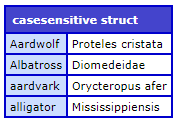### Ordered and case-sensitive structs

Syntax

mystruct = StructNew("ordered-casesensitive")

Example

```<cfscript>
animals=StructNew("ordered-casesensitive")
animals.Aardwolf="Proteles cristata"
animals.aardvark="Orycteropus afer"
animals.alligator="Mississippiensis"
animals.Albatross="Diomedeidae"
writeDump(animals)
</cfscript>```

### Implicit notation

Syntax

```mystruct=\$[
"key1":"val1",
"key2":"val2"
]```

Example

```<cfscript>
animals=\$[
aardwolf:"Proteles cristata",
aardvark:"Orycteropus afer",
alligator:"Mississippiensis",
albatross:"Diomedeidae"
]
writeDump(animals)
</cfscript>```

Output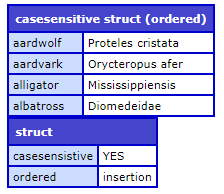## Merge two structs

You can easily merge two struct objects using implicit notation, as well can over-ride the keys in the merged struct.

Example

```<cfscript>

obj1 =\${ key1: 'val1', x: 25 };
obj2 =\${ key2: 'val2', y: 50 };

mergedObj = \$[obj1, obj2, "key3":"val3",z:75]
writeDump(mergedObj);

</cfscript>```

Output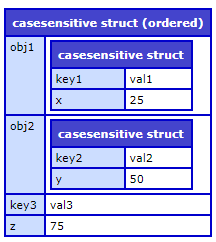## Key override

```<cfscript>
obj1 ={ foo: 'bar', x: 42 };
obj2 ={ foo: 'baz', y: 13 };

mergedObj = {...obj1, ...obj2, "key1":"23"};
writeDump(mergedObj);
</cfscript>```

Output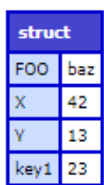## Destructuring Assignment

In ColdFusion, the Destructuring assignment syntax assigns the values from arrays and structs into distinct variables.

The syntax looks similar to array and object literals.

Syntax

`({key1, key2, ............, keyN} = {key1:value1, key2:value2,    , keyN:valueN})`

Using the above statements, you can assign all the variables on the left-hand side to the variables on the right-hand side, with a single line syntax.

The above line is equivalent to writing the following code in ColdFusion:

variable1=value1

Or

key1=value1

For example, using the conventional assignment method, you can write the following snippet:

```<cfscript>
val1=10 val2=20
writeOutput(val1)
writeOutput(val2)
</cfscript>```

Using destructuring assignment, you can rewrite the above as follows:

```<cfscript>
[val1,val2]=[10,20]
writeOutput(val1)
writeOutput(val2)
</cfscript>```

ColdFusion tags also support destructuring. For example,

```<cfset [foo, [[bar], baz]] = [61,[, 23]]>
<cfoutput>#bar#</cfoutput>
<cfoutput>#baz#</cfoutput>```

## Types of destructuring

### Struct destructuring

Struct destructuring allows you to create new variables using an object property as the value.

For example,

```<cfscript>
person = {
name: 'John Doe',
age: 25,
location: {
city: 'Vancouver',
coordinates: [49.2827, -123.1207]
}
};

({name, location: {country, city, coordinates: [lat, lng]},age=40} = person)
</cfscript>
```

Example 2

Nested object destructuring

```<cfscript>
person = {
name: 'John Doe',
age: 25,
location: {
city: 'Vancouver',
coordinates: [49.2827, -123.1207]
}
};

({name, location: {country, city, coordinates: [lat, lng]},age} = person)
writeoutput(name & "<br>");
writeoutput(city & "<br>")
writeoutput(lat & "<br>");
writeoutput(lng & "<br>");
writeoutput(country & "<br>");
writeoutput(age)

</cfscript>```

## Property Shorthand

If you want to define an object whose keys have the same name as the variables passed-in as properties, you can use the shorthand and simply pass the key name.

This syntax gives a cleaner way to define complex structs.

Example 1

```<cfscript>
a = 'foo';
b = 2021;
c = {};

o = {a, b, c}

writeOutput((o.a === 'foo'));
writeOutput((o.b === 2021));
writeDump((o.c));
</cfscript>
```

Output

YESYES

```<cfscript>
CF9 = 'Centaur'
CF10 = 'Zeus'
CF11 = 'Splendor'
CF2016 = 'Raijin'
CF2018 = 'Aether'
CF2021 = 'Project Stratus'

CFReleaseCodeNames = \${
CF9,
CF10,
CF11,
CF2016,
CF2018,
CF2021
}
writeDump(CFReleaseCodeNames);
</cfscript>    ```

Output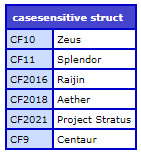Bei Ihrem Konto anmelden